# OAE Mathematics: Applications of Integration Chapter Exam

Exam Instructions:

Choose your answers to the questions and click 'Next' to see the next set of questions. You can skip questions if you would like and come back to them later with the yellow "Go To First Skipped Question" button. When you have completed the practice exam, a green submit button will appear. Click it to see your results. Good luck!

### Page 1

#### Question 1 1. What is the volume made by revolving f(x) around the x-axis between x=0 and x=1?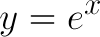#### Question 2 2. If your velocity is given by v(t), and you start at x=4 at t=0, then for what value of t will you be at the position x=20?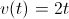#### Question 5 5. What is the equation for the volume of this cone that extends from x=0 to x=A, given that h=x?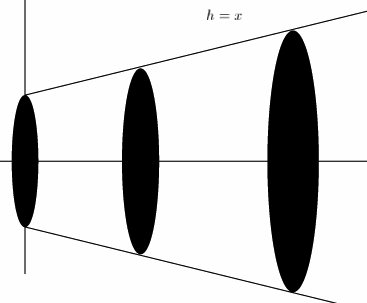### Page 2

#### Question 6 6. What is the volume made by revolving the area between g(x) and f(x) around the x-axis between x=0 and x=1?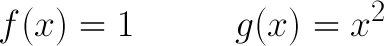#### Question 7 7. Given a velocity v(t) as defined below and a starting position of x=1 at t=0, what is the equation for your position as a function of time?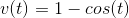#### Question 8 8. Given the cut-off cone pictured, with h = x+3 and the region extending from x=2 to x=4, what is the integral you will need to solve to find the volume of this cut-off cone?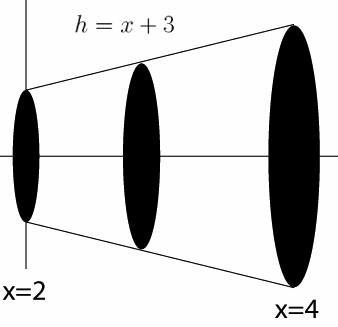#### Question 9 9. What is the equation for the volume of this cone that extends from x=0 to x=A, given that h=x?#### Question 10 10. What is the volume made by revolving f(x) around the x-axis between x=0 and x=1?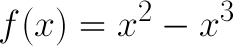### Page 3

#### Question 12 12. Given a velocity of v(t) as defined below, what is the equation for your position as a function of time?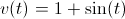#### Question 13 13. What is the volume of the cut-off cone shown?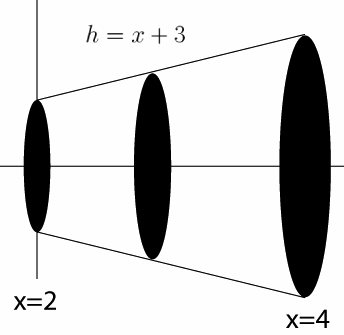#### Question 15 15. What is the equation for the volume of this cone that extends from x=0 to x=A, given that h=x?#### OAE Mathematics: Applications of Integration Chapter Exam Instructions

Choose your answers to the questions and click 'Next' to see the next set of questions. You can skip questions if you would like and come back to them later with the yellow "Go To First Skipped Question" button. When you have completed the practice exam, a green submit button will appear. Click it to see your results. Good luck!

Support